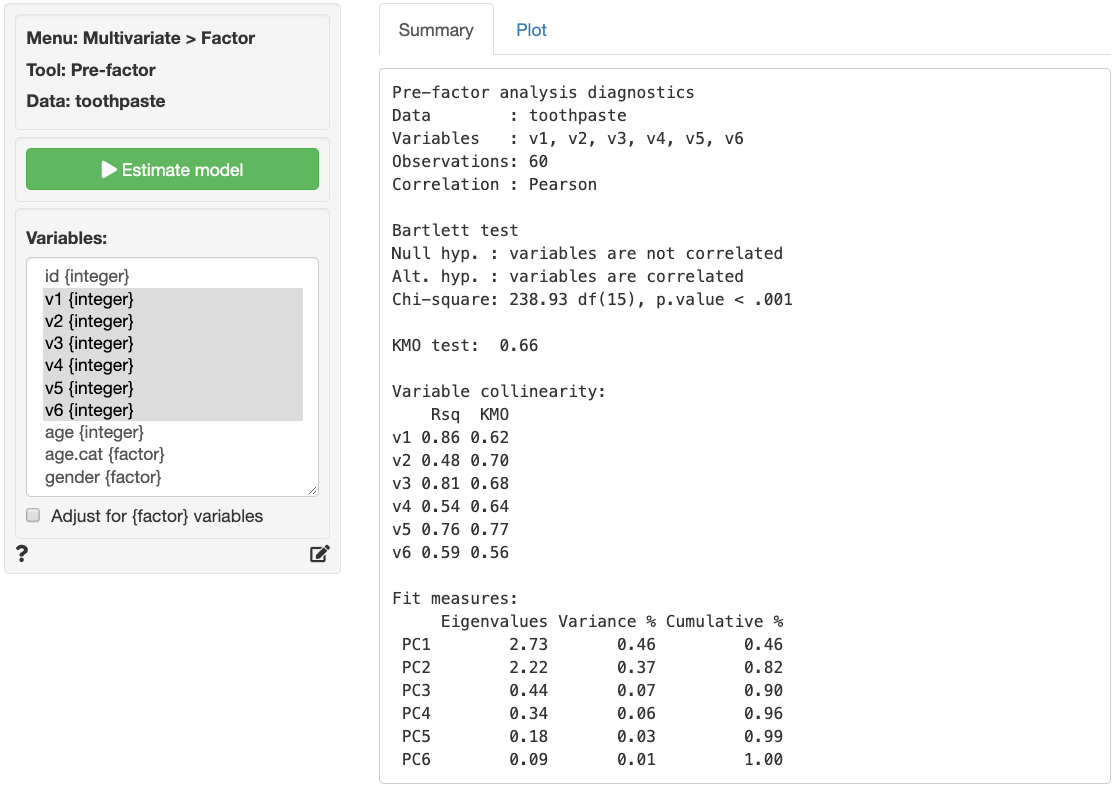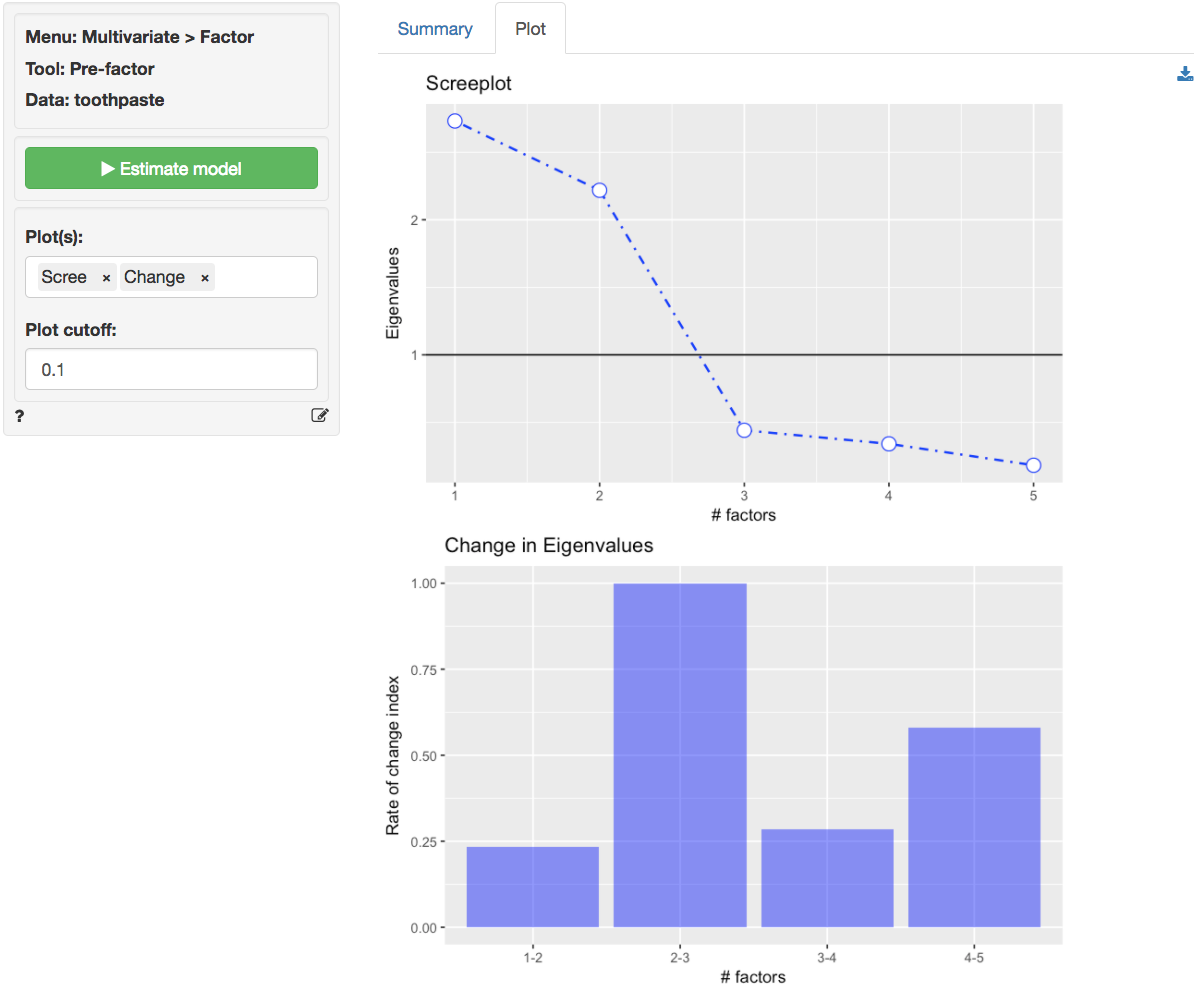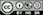Evaluate if data are appropriate for Factor analysis

The goal of Factor Analysis (and Principal Components Analysis) is to reduce the dimensionality of the data with minimal loss of information by identifying and using the structure in the correlation matrix of the variables included in the analysis. The researcher will often try to link the original variables (or items) to an underlying factor and provide a descriptive label for each.

### Example: Toothpaste

First, go to the Data > Manage tab, select examples from the Load data of type dropdown, and press the Load button. Then select the toothpaste dataset. The dataset contains information from 60 consumers who were asked to respond to six questions to determine their attitudes towards toothpaste. The scores shown for variables v1-v6 indicate the level of agreement with statements on a 7-point scale where 1 = strongly disagree and 7 = strongly agree.

The first step in factor analysis is to determine if the data has the required characteristics. Data with limited or no correlation between the variables are not appropriate for factor analysis. We will use three criteria to test if the data are suitable for factor analysis: Bartlett, KMO, and Collinearity for each variable

The KMO and Bartlett test evaluate all available data together. A KMO value over 0.5 and a significance level for the Bartlett’s test below 0.05 suggest there is substantial correlation in the data. Variable collinearity indicates how strongly a single variable is correlated with other variables. Values above 0.4 are considered appropriate. KMO measures can also be calculated for each variable. Values above 0.5 are acceptable.

As can be seen in the output from Multivariate > Factor > Pre-factor below, Bartlett’s test statistic is large and significant (p.value close to 0) as desired. The Kaiser-Meyer-Olkin (KMO) measure is larger than .5 and thus acceptable. The variable collinearity values are above 0.4 and the KMO values are above 0.5 so all variables can be used in the analysis.

To replicate the results shown in the screenshot make sure you have the toothpaste data loaded. Then select variables v1 through v6 and click the Estimate button or press CTRL-enter (CMD-enter on mac) to generate results.The next step is to determine the number of factors needed to capture the structure underlying the data. Factors that do not capture even as much variance as could be expected by chance are generally omitted from further consideration. These factors have eigenvalues < 1 in the output.

A further criteria that is often used to determine the number of factors is the scree-plot. This is a plot of the eigenvalues against the number of factors, in order of extraction. Often a break or elbow is visible in the plot. Factors up to and including this elbow are selected for further analysis if they all have eigenvalues above 1. A set of factors that explain more than 70% of the variance in the original data is generally considered acceptable. The eigenvalues for all factors are shown above. Only two factors have eigenvalues above 1.

At first glance the scree-plot of the Eigenvalues shown below seems to suggest that 3 factors should be extracted (i.e., look for the elbow). The bar plot confirms this insight, i.e., the change in Eigenvalues between factors 1 and 2 is small but the drop-off from 2 to 3 is much larger. However, because the value for the third factor is less than one we will extract only 2 factors.The increase in cumulative % explained variance is relatively small going from 2 to 3 factors (i.e., from 82% to 90%). This is confirmed by the fact that the eigenvalue for factor 3 is smaller than 1 (0.44). Again, we choose 2 factors. The first 2 factors capture 82% of the variance in the original data which is excellent.

## Including categorical variables

The pre-factor analysis diagnostics are calculated using Principal Components Analysis (PCA). The correlation matrix used as input for PCA can be calculated for variables of type numeric, integer, date, and factor. When variables of type factor are included the Adjust for categorical variables box should be checked. When correlations are estimated with adjustment, variables that are of type factor will be treated as (ordinal) categorical variables and all other variables will be treated as continuous.

### Report > Rmd

Add code to Report > Rmd to (re)create the analysis by clicking the icon on the bottom left of your screen or by pressing ALT-enter on your keyboard.

If a plot was created it can be customized using ggplot2 commands or with gridExtra. See example below and Data > Visualize for details.

plot(result, plots = "scree", custom = TRUE) +
labs(caption = "Data used from ...")

### R-functions

For an overview of related R-functions used by Radiant to conduct factor analysis see Multivariate > Factor.

The key functions used in the pre_factor tool are cor from the stats package, eigen from base, and cortest.bartlett and KMO from the psych package.

© Vincent Nijs (2019)